# GRE Subject Test: Math : Complements

## Example Questions

### Example Question #11 : Relations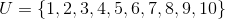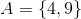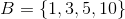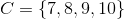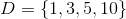What is the value of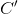?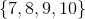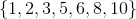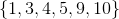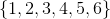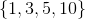Explanation:

When you see a letter with the prime symbol, that means complement. Complement tells you to take whatever is NOT in that set and what is in the universal set. You could also say you are eliminating the numbers in the universal set that are in the set you are complementing.

The universal set isSetTaking the numbers 7, 8, 9, and 10 out of the universal set you get the answer### Example Question #21 : Real Analysis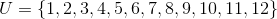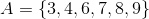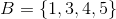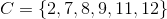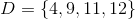Evaluate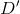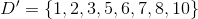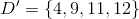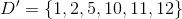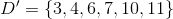Explanation:

The prime symbol means to take the complement of set D. To find a complement, you want to only include the numbers in the universal set that do not appear in the set being complemented.

If setand the universal set isThe numbers you want to include are everything in the universal set except 4, 9, 10, 11, and 12

This leaves the numbers### Example Question #2 : ComplementsEvaluate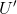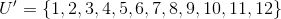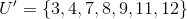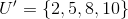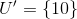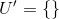Explanation:

A complement symbol means you want to only include the numbers in the universal set that do not appear in the set being complemented.

However, all the numbers in the universal set appear in itself. This means the complement of ANY universal set is the empty set.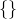### Example Question #4 : ComplementsEvaluate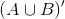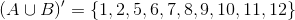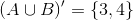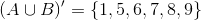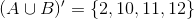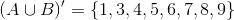Explanation:Always work in parentheses first. First, you need to solve the union of sets A and B. Union means to include everything in both sets without repeating any duplicates.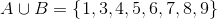Now take the complement of this by including the numbers in the universal set that do not appear in A union B. This leaves### Example Question #5 : Complements

If angle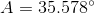, find angleif anglesandare complementary.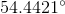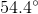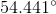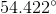Explanation:

Step 1: Recall the definition of Complementary angles.

Two angles are complementary if the sum of the two angles is ALWAYS.

Step 2: If the sum of the angles is, then we can subtract the other one to find the missing angle...

So,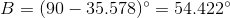### All GRE Subject Test: Math Resources